# Test: Three Dimensional 3D Geometry (Competition Level) - 2

## 25 Questions MCQ Test Mathematics (Maths) Class 12 | Test: Three Dimensional 3D Geometry (Competition Level) - 2

Description
This mock test of Test: Three Dimensional 3D Geometry (Competition Level) - 2 for JEE helps you for every JEE entrance exam. This contains 25 Multiple Choice Questions for JEE Test: Three Dimensional 3D Geometry (Competition Level) - 2 (mcq) to study with solutions a complete question bank. The solved questions answers in this Test: Three Dimensional 3D Geometry (Competition Level) - 2 quiz give you a good mix of easy questions and tough questions. JEE students definitely take this Test: Three Dimensional 3D Geometry (Competition Level) - 2 exercise for a better result in the exam. You can find other Test: Three Dimensional 3D Geometry (Competition Level) - 2 extra questions, long questions & short questions for JEE on EduRev as well by searching above.
QUESTION: 1

### Equation of plane which passes through the point of intersection of l ines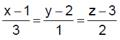and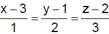and at greatest distance from the point (0, 0, 0) is

Solution:

x = 3λ + 1, y = λ + 2 and 2λ + 3 x = m + 3, y = 2m + 1 and 3m + 2 Lines intersect.
Therefore 3λ + 1 = m + 3 and  λ + 2 = 2λ + 1
3λ - u - 2 = 0
λ - 2u + 1 = 0
Apply ‘2312’ we get
λ/(-1-4) + u(-2-3) = 1(-6+1)
λ = 1 and u = 1
Therefore, point of intersection is (4, 3, 5).
Now plane passing through (4, 3, 5) and at maximum distance from the origin must have directions of the normal as 4 − 0, 3 − 0 and 5 − 0.
Therefore, equation of required plane is (x − 4)4 + (y − 3)3 + (z − 5)5 = 0 or  4x + 3y + 5z = 16 + 9 + 25 ⇒ 4x + 3y + 5z = 50

QUESTION: 2

Solution:
QUESTION: 3

### In the adjacent figure ‘P’ is any arbitrary interior point of the triangle ABC such that the lines AA1,BB1,CC1 are concurrent at P. Value of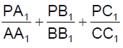is always equal to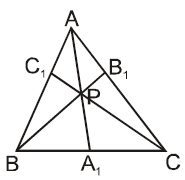Solution:
QUESTION: 4

A tetrahedron has vertices at O(0, 0, 0), A(1, 2, 1), B(2, 1, 3) and C(–1, 1, 2). Then the angle between the face OAB and ABC will be

Solution:
QUESTION: 5

The two lines x = ay + b, z = cy + d and = a' y + b', z = c' y + d' will be perpendicular, iff

Solution:
QUESTION: 6

ABC is a triangle where A = (2, 3, 5), B = (–1, 2, 2) and C(λ, 5, μ). If the median through A is equally inclined to the axes then

Solution:
QUESTION: 7

A mirror and a source of light are situated at the origin O and at a point on OX, respectively. A ray of light from the source strikes the mirror and is reflected. If the D.r.’s of the normal to the plane are 1, –1, 1, then D.C.’s of the reflected ray are

Solution:
QUESTION: 8

The equation of motion of a point in space is x = 2t, y = –4t, z = 4t where t measured in hours and the co-ordinates of moving point in kilometers. The distance of the point from the starting point O(0, 0, 0) in 10 hours is

Solution:
QUESTION: 9

Minimum value of x2 + y2 + z2 when ax+by+cz = p is

Solution:
QUESTION: 10

The direction cosines of a line equally inclined to three mutually perpendicular lines having ℓ1, m1, n1, ; ℓ2, m2, n2 ; ℓ3, m3, n3 are

Solution:
QUESTION: 11

The co-ordinates of the point where the line joining the points (2, –3, 1), (3, –4, –5) cuts the plane 2x + y + z = 7 are

Solution:
QUESTION: 12

If the line joining the origin and the point (–2, 1, 2) makes angle θ1 ,θand θ3 with the positive direction of the coordinate axes, then the value of cos 2θ1 + cos 2θ2 + cos 2θ3 is

Solution:
QUESTION: 13

The square of the perpendicular distance of point P(p, q, r) from a line through A(a, b, c) an whose direction cosine are (ℓ) , m, n is

Solution:
*Multiple options can be correct
QUESTION: 14

Equation of the plane passing through A(x1, y1, z1) d containing the line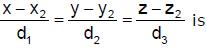Solution:
*Multiple options can be correct
QUESTION: 15

The equation of the line x + y + z – 1 = 0, 4x + y – 2z + 2 = 0 written in the symmetrical form is

Solution:
*Multiple options can be correct
QUESTION: 16

The acute angle that the vector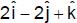makes with the plane contained by the two vectors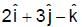and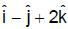is given by

Solution:
*Multiple options can be correct
QUESTION: 17

The ratio in which the sphere x2 + y2 + z2 = 504 divides the line joining the points (12, –4, 8) and (27, –9, 18) is

Solution:
*Multiple options can be correct
QUESTION: 18

The equation of the planes through the origin which are parallel to the line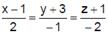and distance 5/3 from it are

Solution:
*Multiple options can be correct
QUESTION: 19

If the edges of a rectangular parallelopiped are 3, 2, 1 then the angle between a pair of diagonals is given by

Solution:
*Multiple options can be correct
QUESTION: 20

Consider the lines x/2 = y/3 = z/5 and x/1 = y/2 = z/3 equation of the line which

Solution:
*Multiple options can be correct
QUESTION: 21

The direction cosines of the lines bisecting the anglebetween the lines whose direction cosines are ℓ1, m1, n1 and ℓ2, m2, n2 and the angle between these lines is 0, are

Solution:
*Multiple options can be correct
QUESTION: 22

The equation og line AB is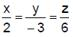. Through a point P(1, 2, 5), line PN is drawn perpendicular to AB and line PQ is drawn parallel to the plane 3x + 4y + 5z = 0 to meet AB is Q. Then

Solution:
*Multiple options can be correct
QUESTION: 23

The planes 2x – 3y – 7z = 0, 3x – 14y – 13z = 0 and 8x – 31y – 33z = 0

Solution:
*Multiple options can be correct
QUESTION: 24

If the length of perpendicular drawn from origin on a plane is 7 units and its direction ratios are –3, 2, 6, then that plane is

Solution:
*Multiple options can be correct
QUESTION: 25

Let a perpendicular PQ be drawn from P(5, 7, 3) to the line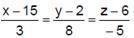when Q is the foot.
Then

Solution: